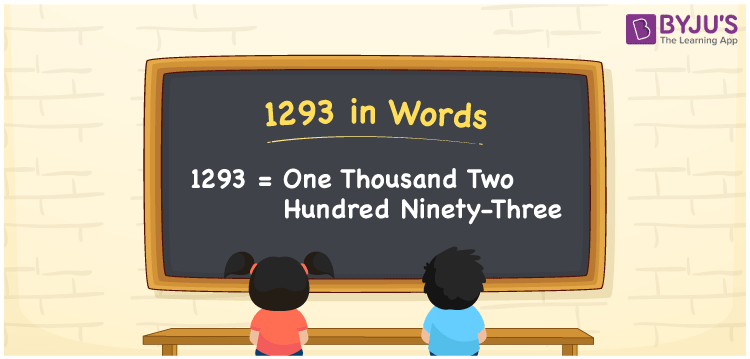# 1293 in Words

Suppose you have written 1293 words to narrate a story, then you can say, “I narrated a story in One thousand two hundred ninety-three words”. Thus, we can express the number 1293 in words as One thousand two hundred ninety-three. Let’s understand how to convert the cardinal number 1293 into words here in this article.

 1293 in words One thousand two hundred ninety-three One thousand two hundred ninety-three in Numbers 1293

## 1293 in English Words

Generally, we express numbers in words using the English alphabet. Thus, we spell 1293 in English as “One thousand two hundred ninety-three”.## How to Write 1293 in Words?

As we can see, 1293 is a four-digit number so, let’s create a four-column place value chart as shown below.

 Thousands Hundreds Tens Ones 1 2 9 3

Here, ones = 3, tens = 9, hundreds = 2, and thousands = 1.

These digits can be expanded as:

1 × Thousand + 2 × Hundred + 9 × Ten + 3 × One

= 1 × 1000 + 2 × 100 + 9 × 10 + 3 × 1

= 1000 + 200 + 90 + 3

= One thousand + Two hundred + Ninety + Three

= One thousand two hundred ninety-three

Therefore, 1293 in words = One thousand two hundred ninety-three.

1293 is a natural number that precedes 1294 and succeeds 1292.

1293 in words – One thousand two hundred ninety-three

Is 1293 an even number? – No

Is 1293 an odd number? – Yes

Is 1293 a composite number? – Yes

Is 1293 a prime number? – No

Is 1293 a perfect square number? – No

Is 1293 a perfect cube number? – No

## Frequently Asked Questions on 1293 in Words

Q1

### Write 1293 in words.

We can write the number 1293 in words as One thousand two hundred ninety-three.
Q2

### How to write Rs. 1293 in words on a cheque?

On a cheque, we generally write Rs. 1293 in words as “One thousand two hundred ninety-three rupees only”.
Q3

### What is the number name of 1293?

The number name of 1293 is One thousand two hundred ninety-three.